# Composite Functions

In this article, we you will learn about composite functions, it means that a function can become input of another function. Before you learn about the composite functions, you must be familiar with the arithmetic of functions which means given two function , you will be able to perform basic arithmetic operations on the function itself. Identifying Domain

Sometimes the function expression is given, but domain is not specified. In such cases, you must identify the domain of given function. Suppose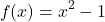is a function whose domain is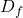which is “all real numbers”, because the function is true for all real numbers. Another function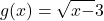has a domain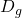which must have all numbers that are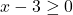otherwise the function is invalid. Therefore,contains all numbers in the interval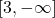.

Why this is important ? because when we perform arithmetic operations on two or more functions the domain of new function is set of all real numbers that belong to bothand, that is,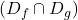.

### Arithmetic Operations on Functions

Normal arithmetic operations are possible on functions too. If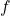and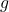are two functions, then there are four operations possible on these functions.

1. Sum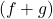2. Difference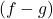3. Product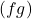4. Quotient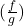Now we discuss about each of these operations.

Sum of functions

The sum of functions is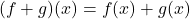The expressions of both functions are added together to form a new function. For example, if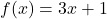and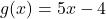, then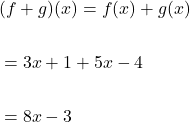Difference of functions

The difference of functions is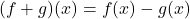The expressions of functionis subtracted from expression of functionto form a new expression for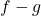. For example, ifand, then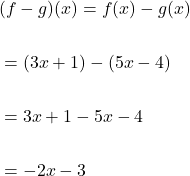Product of functions

The product of the functions is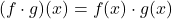The expressions of functionandis multiplied to get the new product expression of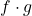. Each term is ofis multiplied with each term of. For example, if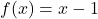and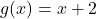are two expressions, then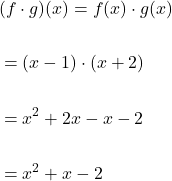Quotient of functions

The quotient of functions is obtained by dividing two functions, which is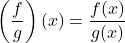The functions are divided to get the quotient, however, there is one condition, that is,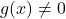, otherwise the quotient of function will be “divide by 0” which is “undefined“.

Therefore, if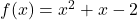and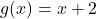, then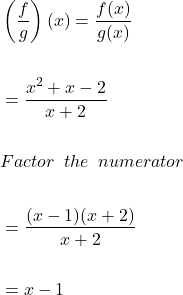The domain of,,is set of all reals numbers that are common to domain of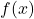and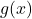which isexcept wherefor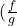.

### Composite Functions

The composite functions or the idea of composition of functions is simple. Suppose there are two functions,and, then if functionbecomes input for function, it is called “composition of functionwith functionor simply a composite function.

Let us try to understand this with an example, suppose Nancy work as a maid, and get paid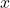amount every week and after paying taxes she receives only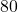of her earnings. Each week she spends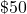for grocery from her earning . The total earning of Nancy after paying taxes can be defined by function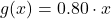. Her savings after expense of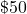can be defined as function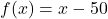.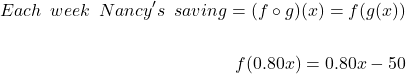If her gross earning is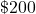, then her saving would be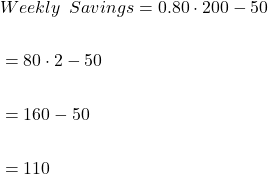Therefore, total saving after paying taxes and expenses ofis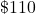.

What is the domain of a composite function?

If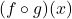is a composite function such that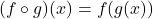. Then the domain of composite function must be

1.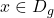meaning “must be in the domain of.
2.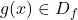meaning “must be in the domain of.

Therefore, while finding the domain of a composite function, we must first exclude all values ofthan can make the function“invalid”. Also, ifhappens to be valid, that is,, thenmust be valid value for the function. Ifhappens to invalid value, then bothandmust be excluded from the domain of composite function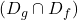.

### Decomposing Functions

Like composing two functions, it is possible to decompose a function because we know that “composition of two functions” creates a new function. Consider the following example,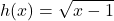Here we can clearly see that there are two functions involved in the expression. Therefore,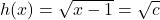where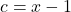which implies that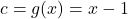.

We can write the function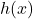andas composition of function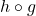where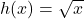and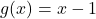.